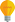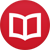# Integral of $\sin(x)\cos(x)$

by Jakub MarianTip: See my list of theMost Common Mistakes in English. It will teach you how to avoid mis­takes with com­mas, pre­pos­i­tions, ir­reg­u­lar verbs, and much more.

The function $\sin(x)\cos(x)$ is one of the easiest functions to integrate. All you need to do is to use a simple substitution $u = \sin(x)$, i.e. $\frac{du}{dx} = \cos(x)$, or $dx = du/\cos(x)$, which leads to

$$∫ \sin(x)\cos(x)\,dx = \l.∫ u\,du\r|_{u = \sin(x)} = \frac{u^2}2 + C = \frac12 \sin^2(x)+C\,.$$

Another way to integrate the function is to use the formula

$$\sin(2x) = 2\sin(x)\cos(x) \quad ⇒ \quad \sin(x)\cos(x) = \frac12 \sin(2x)\,$$

so

$$∫ \sin(x)\cos(x)\,dx = \frac12 ∫ \sin(2x)\,dx = -\frac14 \cos(2x)+C$$

It is worth mentioning that the $C$ in the equality above is not the same $C$ as in our original expression. In fact, it is possible to calculate that $\frac12 \sin^2(x) - (-\frac14 \cos(2x)) = 1/4$, so the two solutions lead to the same result, just shifted by a constant.

By the way, I have written several educational ebooks. If you get a copy, you can learn new things and support this website at the same time—why don’t you check them out?